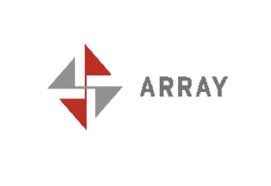1. Do array subscripts always start with zero?

Yes. If you have an array a[MAX] (in which MAX is some value known at compile time), the first element is a, and the last element is a[MAX-1]. This arrangement is different from what you would find in some other languages. In some languages, such as some versions of BASIC, the elements would be a through a[MAX], and in other languages, such as Pascal, you can have it either way.
This variance can lead to some confusion. The “first element” in non-technical terms is the “zero’th” element according to its array index. If you’re using spoken words, use “first” as the opposite of “last.” If that’s not precise enough, use pseudo-C. You might say, “The elements a sub one through a sub eight,” or, “The second through ninth elements of a.”

Because pointers and arrays are almost identical, you might consider creating a pointer that would refer to the same elements as an array but would use indices that start with one. For example:

/* don’t do this!!! */

int     a0[ MAX ];

int     *a1 = a0 – 1;   /* & a[ -1 ] */

Thus, the first element of a0 (if this worked, which it might not) would be the same as a1. The last element of a0, a0[MAX-1], would be the same as a1[MAX]. There are two reasons why you shouldn’t do this.

The first reason is that it might not work. According to the ANSI/ISO standard, it’s undefined (which is a Bad Thing). The problem is that &a[-1] might not be a valid address; Your program might work all the time with some compilers, and some of the time with all compilers. Is that good enough?

The second reason not to do this is that it’s not C-like. Part of learning C is to learn how array indices work. Part of reading (and maintaining) someone else’s C code is being able to recognize common C idioms. If you do weird stuff like this, it’ll be harder for people to understand your code. (It’ll be harder for you to understand your own code, six months later.)

2. Is it valid to address one element beyond the end of an array?

It’s valid to address it, but not to see what’s there. (The really short answer is, “Yes, so don’t worry about it.”) With most compilers, if you say

int i, a[MAX], j;

then either i or j is at the part of memory just after the last element of the array. The way to see whether i orj follows the array is to compare their addresses with that of the element following the array. The way to say this in C is that either

& i == & a[ MAX ]

is true or

& a[ MAX ] == & j

is true. This isn’t guaranteed; it’s just the way it usually works. The point is, if you store something in a[MAX], you’ll usually clobber something outside the a array. Even looking at the value of a[MAX] is technically against the rules, although it’s not usually a problem. Why would you ever want to say &a[MAX]? There’s a common idiom of going through every member of a loop using a pointer. Instead of

for ( i = 0; i < MAX; ++i )

{

/* do something */;

}

C programmers often write this:

for ( p = a; p < & a[ MAX ]; ++p )

{

/* do something */;

}